RD Sharma Solutions Ex-24.2, Measures Of Central Tendency, Class 9, Maths

# RD Sharma Solutions Ex-24.2, Measures Of Central Tendency, Class 9, Maths - Notes | Study RD Sharma Solutions for Class 9 Mathematics - Class 9

 1 Crore+ students have signed up on EduRev. Have you?

Q 1 . Calculate the mean for the following distribution :

 x : 5 6 7 8 9 f : 4 8 14 11 3

SOLUTION :

 x f fx 56789 4814113 2048988827 N=40 ∑fx = 281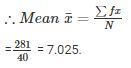Q 2 . Find the mean of the following data :

 x : 19 21 23 25 27 29 31 f : 13 15 16 18 16 15 13

SOLUTION :

 x f fx 19212325272931 13151618161513 247315368450432435403 N=106 ∑fx=2650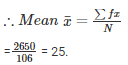Q 3 . The mean of the following data is 20.6 .Find the value of p.

 x : 10 15 p 25 35 f : 3 10 25 7 5

SOLUTION :

 x f fx 1015P2535 3102575 3015025p175175 N = 50 ∑fx=25p+530

It is given that ,

Mean = 20.6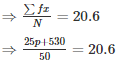⇒ 25p+530 = 20.6×50

⇒ 25p = 1030−530

⇒ 25p = 500

⇒ p = 50025 = 20

⇒ p = 20

∴ p = 20.

Q 4 . If the mean of the following data is 15 , find p.

 x : 5 10 15 20 25 f : 6 p 6 10 5

SOLUTION :

 x f fx 510152025 6P6105 3010p90200125 N = p+27 ∑fx = 10p+445

It is given that ,

Mean = 15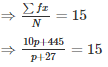⇒ 10p+445 = 15×(p+27)

⇒10p+445 = 15p+405

⇒15p−10p = 445−405

⇒ 5p = 40

⇒ p = 40/5 =8

⇒ p = 8

∴ p = 8.

Q 5. Find the value of p for the following distribution whose mean is 16.6.

 x : 8 12 15 p 20 25 30 f : 12 16 20 24 16 8 4

SOLUTION :

 x f fx 81215P202530 121620241684 9619230024p320200120 N = 100 ∑fx = 24p+1228

It is given that ,

Mean = 16.6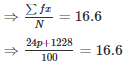⇒ 24p+1228 = 1660

⇒ 24p = 1660−1228

⇒ 24p = 432

⇒ p = 432/24 = 18

⇒ p = 18

∴ p = 18.

Q 6 . Find the missing value of p for the following distribution whose mean is 12.58 .

 x : 5 8 10 12 p 20 25 f : 2 5 8 22 7 4 2

SOLUTION :

 x f fx 581012P2025 25822742 1040802647p8050 N = 50 ∑fx=7p+524

It is given that ,

Mean = 12.58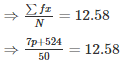⇒ 7p+524 = 629

⇒ 7p = 629−524

⇒ 7p = 105

⇒ p = 1057 = 15

⇒ p = 15

∴ p = 18.

Q7 . Find the missing frequency (p) for the following distribution whose mean is 7.68 .

 x : 3 5 7 9 11 13 f : 6 8 15 p 8 4

SOLUTION :

 x f fx 35791113 6815P84 18401059p8852 N=p+41 ∑fx=9p+303

It is given that ,

Mean = 7.68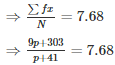⇒ 9p+303 = 7.68p+314.88

⇒ 9p−7.68p = 314.88−303

⇒ 1.32p = 11.88

⇒ p = 11.881.32 = 9

⇒ p = 9

∴ p = 9.

Q 8. Find the value of p, if the mean of the following distribution is 20 .

 x : 15 17 19 20+p 23 f: 2 3 4 5p 6

SOLUTION :

 x f fx 15171920+p23 2345p6 305176100p+ 5p2138 N=5p+15 fx= 5p2+100p+295

It is given that ,

Mean = 20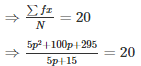⇒ 5p2+100p+295 = 20(5p+15)

⇒ 5p2+100p+295 = 100p+300

⇒ 5p2 =300−295

⇒ 5p2 = 5

⇒ p2 = 1

⇒ p = ±1

Frequency can’t be negative.

Hence, value of p is 1.

Q 9 . Find the mean of the following distribution :

 x : 10 12 20 25 30 f : 3 10 15 7 5

SOLUTION :

 x f fx 1012202535 3101575 30120300175175 N = 40 ∑fx = 800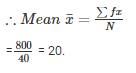Q 10. Candidates of four schools appear in a mathematics test. The data were as follows :

 Schools No. Of Candidates Average Score IIIIIIIV 6048Not Available40 75805550

If the average score of the candidates of all four schools is 66 , Find the number of candidates that appeared from school III .

SOLUTION :

 Schools No. Of Candidates Average Score IIIIIIIV 6048x40 75805550

Given the average score of all schools = 66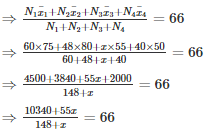⇒ 10340+55x = 66x+9768

⇒ 10340−9768 = 66x−55x

⇒ 11x = 572

⇒ x = 572/11 = 52

∴ No. of candidates appeared from school III = 52.

Q.11 . Five coins were simultaneously tossed 1000 times and at each, toss the number of heads was observed. The number of tosses during which 0 , 1 , 2 , 3 , 4 and 5 heads were obtained are shown in the table below. Find the mean number of heads per toss.

 No . of heads per toss No.of tosses 012345 3814434228716425 Total 1000

SOLUTION :

 No . of heads per toss(x) No.of tosses(f) fx 012345 3814434228716425 0144684861656125 N = 1000 ∑fx = 2470

∴ Mean number of heads per toss

= 2470/1000

= 2.47

Q 12 . Find the missing frequencies in the following frequency distribution if it is known that the mean of the distribution is 50.

 x : 10 30 50 70 90 f : 17 f1 32 f2 19

Total = 120

SOLUTION :

 x f fx 10 30507090 17 f132f219 170 30f1160070f21710 N=120 ∑fx = 3480+30f1+70f2

It is given that

Mean = 50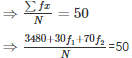⇒ 3480+30f1+70f= 50×120

⇒ 30f1+70f= 6000−3480

⇒10(3f1+7f2) = 10(252)

⇒3f1+7f= 252⋅⋅⋅⋅⋅(1)                         [∵Divideby10]

And N = 20

⇒17+f1+32+f2+19 = 120

⇒ 68+f1+f= 120

⇒ f1+f= 120−68

⇒ f1+f= 52

Multiply with 3 on both sides

⇒3f1+3f= 156⋅⋅⋅⋅⋅⋅(2)

Subtracting equation (2) from equation (1)

⇒3f1+7f2−3f1−3f= 252−156

⇒ 4f2=96

⇒ f= 96/4 = 24

Put the value of f2 in equation (1)

⇒ 3f1+7×24 = 252

⇒ 3f= 252−168

⇒ f= 84/3 = 28

⇒ f= 28

The document RD Sharma Solutions Ex-24.2, Measures Of Central Tendency, Class 9, Maths - Notes | Study RD Sharma Solutions for Class 9 Mathematics - Class 9 is a part of the Class 9 Course RD Sharma Solutions for Class 9 Mathematics.
All you need of Class 9 at this link: Class 9

## RD Sharma Solutions for Class 9 Mathematics

91 docs
 Use Code STAYHOME200 and get INR 200 additional OFF

## RD Sharma Solutions for Class 9 Mathematics

91 docs

Track your progress, build streaks, highlight & save important lessons and more!

,

,

,

,

,

,

,

,

,

,

,

,

,

,

,

,

,

,

,

,

,

,

,

,

,

,

,

,

,

,

;